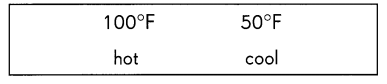# Math in Focus Grade 3 Chapter 16 Practice 7 Answer Key Real-World Problems: Time and Temperature

This handy Math in Focus Grade 3 Workbook Answer Key Chapter 16 Practice 7 Real-World Problems: Time and Temperature provides detailed solutions for the textbook questions.

## Math in Focus Grade 3 Chapter 16 Practice 7 Answer Key Real-World Problems: Time and Temperature

Solve.

Question 1.
A watch is 15 minutes slow. It shows 12:50 A.M. What is the actual time?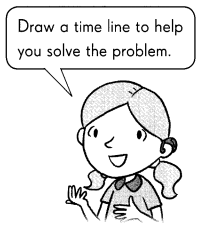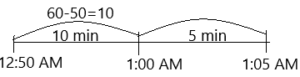Explanation:
1. I labelled 12:50 AM on the timeline.
2. I subtracted 50 min of 12:50 from the 60 minutes. then I get 60-50=10 min. The 10 min from 12:50 is 10:00 AM.
3. In the question given was 15 minutes slow. Actually, we calculated 10 minutes and the remaining 5 minutes need to calculate.
4. From 1:00 Am the 5 minutes are 1:05 AM.
5. Therefore, the actual time is 1:05 AM.

Question 2.
A clock is 20 minutes fast. It shows 9:35 P.M. What is the actual time?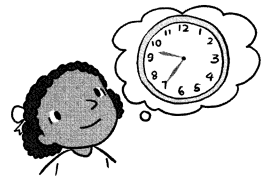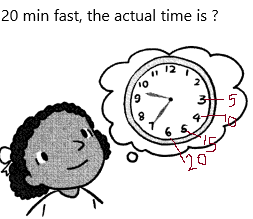Explanation:
1. The time is showing 9:35 PM which is 20 min fast.
2. We need to calculate the actual time. Once watch the above diagram in which we represented the minutes.
3. Count from the 6 which is 20 (multiple of 5), 5 as 15, 4 as 10, 3 as 5.
4. Now the actual time will be 3 as 9:15, 4 as 9:20, 5 as 9:25, 6 as 9:30.
5. The time is showing 20 minutes faster so the actual time is 9:15 PM.

Question 3.
Joseph takes 50 minutes to install a software program. How long does he take to install 4 such software programs? Give your answer in hours and minutes.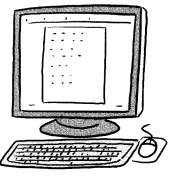The minutes’ Joseph takes to install a software=50
The time he will take if he installs 4 softwares=X
Now calculate the value of X.
X=4*50=200 minutes.
200 minutes we can say in hours and minutes are 3 hours 20 minutes.
200 can be divided into 180 and 20
If we want to convert minutes to hours then divide with 60.
=180/60
=3.
And the remaining 20 min will stay the same.
The total time he takes to install 4 software is 3 h 20 min.

Question 4.
Mr. Sperando left home at 6:35 A.M. He took 1 hour 5 minutes to travel to his office. What time did he reach his office?Starting time to office=6:35 AM
The reaching time to office=X
The elapsed time=1 h 5 min.
By using the start time and elapsed time we can calculate the reaching time of office.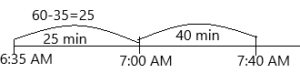Explanation:
1. I labelled starting time on the timeline.
2. By using the elapsed time I calculated ending time. I subtracted 35 min of 6:35 from the 60 min to get 25 min.
3. From 6:35 the 25 min of time is 7:00 AM. We need to calculate for 1 h 5 min, already we calculate 25 min extra 40 min we have to calculate.
4. we already know 1 hour=60 minute. By keeping this thing in mind we need to calculate. So we got 25 min in 1 h 5 min. 1 h 5 min=65 min. To get the remaining min, subtract 25 from 65 then we get 65-25=40. Now calculate for 40 min.
5. From 7:00 the 40 min will be 7:40 AM.
6. The ending time means the value of X is 7:40 AM.

Question 5.
Elaine spends 5 hours 30 minutes in school. If she reaches school at 9:00 A.M. What time does she leave school?The elapsed time=5 h 30 min
Starting time=9:00 AM
The ending time of school=X.
By using the start time and elapsed time we can calculate the ending time of school.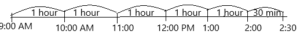Explanation:
1. I labelled starting time on the timeline.
2. Between 9:00 AM to 10:00 AM there is 1 hour.
3. Between 10:00 AM to 11:00 AM there is 1 hour.
4. Between 11:00 AM to 12:00 PM there is 1 hour.
5. Between 12:00 PM to 1:00 PM there is 1 hour.
6. Between 1:00 PM to 2:00 PM there is 1 hour.
7. The hours are 9:00 to 2:00 is 10:00, 11:00, 12:00. 1:00. Totally, 5 hours.
8. Now we need to calculate for 30 minutes. From 2 the 30 min means 2:30
9. So the ending time of school X=2:30 PM.

Question 6.
Heather has a dance lesson after school. School ends at 2:55 P.M. It takes Heather 1 5 minutes to walk to her dance studio. After her 1 hour lesson, it takes Heather 15 minutes to walk home. What time does Heather get home?
The time the school ends=2:55 PM
The time is taken to walk to her dance school from school=15 min
The time she spends on dance school=1 hours
The time she goes home by walk from her dance school=15 min.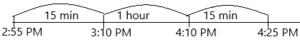Explanation:
1. Calculate by using the given timings.
2. Draw a timeline for better understanding. Once observe the above timeline.
3. The time 2:55, after 15 min the time is 3:10 PM.
4. She reached dance school by walk is 3:10 PM
5. She takes 1 hour in the dance school that is 4:10 PM
6. Now she is going to home on the walk which takes 15 min. So the time after 15 min is 4:25 PM.

Question 7.
Derek takes 30 minutes to make one friendship bracelet.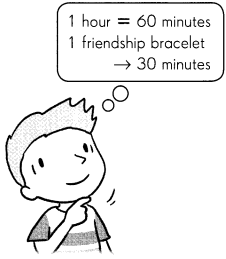a. How many hours does he take to make 8 similar bracelets?
Explanation:
The number of bracelets he wants to make=8.
The time will take to complete 8 bracelets=X.
We already know 1 hour=60 min
In the question given for making 1 bracelet 30  minutes are taken.
X=8*30
X=240 min
To convert min to hours then divide 240 by 60.
X=240/60
X=4 hours.
Therefore, 4 hours will take to complete 8 bracelets.

b. How many similar bracelets can he make in 10 hours?
We can calculate this by using cross multiplication.
for making 1 bracelet 30 minutes are taken.
For 10 bracelets, we need to calculate.
To calculate in hours convert min to hours.
1 hour = 60 min
if we make 2 bracelets, 1 hour will be taken.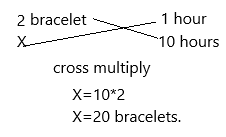Therefore, in 10 hours 20 bracelets we could make.

Question 8.
Hannah left home at 6:15 A.M. She took 1 hour 55 minutes to ride to Maple Park and 2 hours 20 minutes to ride home. She did not spend any time at the park.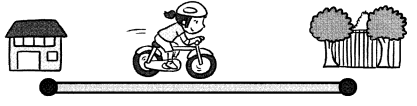a. How long did she spend riding in all?
Explanation:
– If the minutes are 60 or more, subtract 60 from the minutes and add 1 to hours
The timings are: 1 h 55 min and 2 h 20 min
= 1+2
=3
=55+20
=75
Step 3:The minutes are >60, then subtract 60 from the minutes and 1 to the hours and do the same process.
=60-55
=5 min
Now by combining these two we get 1 h 5 min.
Now calculate for the 2 h 5 min and 2 h 20 min:
By combing steps 4 and step 5 we get the time that is 4 hours 25 minutes.
Therefore, 4 h 25 min she spends on riding.

b. What time did she return home?
The starting time=6:15 AM
The elapsed time =2 h 20 min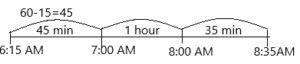The time she returned to home is 8:35 AM

Question 9.
Twyla sews teddy bears. She takes 3 hours 40 minutes to sew the first teddy bear and 2 hours 55 minutes to sew the second teddy bear. She finishes sewing the teddy bears at 8:10 P.M. What time did she start sewing?The time is taken for the first teddy to sew=3 h 40 min
The time is taken to sew the second teddy=2 h 55 min
The ending time is 8:10 PM
– If the minutes are 60 or more, subtract 60 from the minutes and add 1 to hours
The timings are: 3 h 40 min and 2 h 55 min
= 3+2
=5
=55+40
=95.
Step 3:The minutes are >60, then subtract 60 from the minutes and 1 to the hours and do the same process.
=60-40
=20 min
Now by combining these two we get 4 h 20 min.
Now calculate for the 4 h 20 min and 2 h 20 min:
By combing steps 4 and step 5 we get the time that is 4 hours 25 minutes.
Therefore, 6 h 40 min to sew total teddys.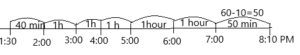By applying elapsed time concept we have to calculate the starting time.
The time started for sewing teddys are 1:30 PM

Question 10.
Jim finished work at 5:10 P.M. according to his watch. His watch was 15 minutes slow.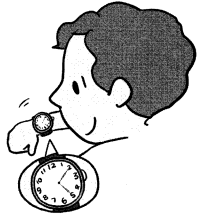a. What was the actual time he finished work?
The actual time is 5:25 PM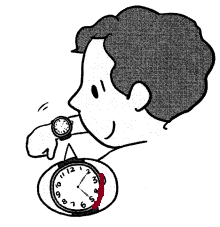b. He worked for 8 hours 15 minutes. What was the actual time he started work?
We take the finished work above 5:25 PM (a).
The elapsed time is 8 h 15 min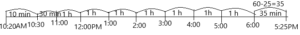Once observe the timeline above.
By applying elapsed time rules:
The actual work starts at 10:20 AM.

Complete each story. Use the temperatures and words in the box.

Question 11.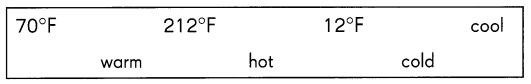It was winter and the temperature outside was 12 degrees Fahrenheit. It was very cold outside. Kate had set the heater to 70 degrees Fahrenheit and it was warm in the house. She put a kettle of water to boil and the temperature of the water was 212 degrees Fahrenheit. The water was hot enough to make a hot drink.